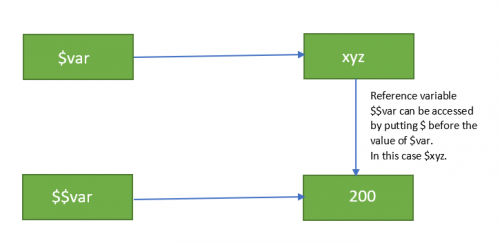# PHP \$ and \$\$ variables

In this tutorial, you will learn about the differences between \$var and \$\$var in PHP.

The \$var(Single Dollar) is the normal PHP variable with the name var that stores numeric and non-numeric values like integer, double, string, etc.

The \$\$var(Double variable) is the reference variable that stores the value of \$var into it.

This enables you to have a variable’s variable and store the reference of another variable. Without much delay, let’s understand the difference with the help of some examples.

### Example 1: PHP \$ and PHP \$\$

```<?php
\$var = "xyz";
\$\$var = 200;
echo \$var."<br/>";
echo \$\$var."<br/>";
echo \$xyz;
?>```

Output

xyz
200
200

In the above example, we have assigned a variable \$var with the value `xyz` and reference variable \$\$var assigned with 200. Now, we have printed the value of \$var, \$\$var, and \$xyz.

#### Note: Reference variable \$\$var can be accessed by putting \$ before the value of \$var. In this case \$xyz.### Example 2

```<?php
\$x = "Maharashtra";
\$\$x = "Mumbai";
echo \$x."<br/>";
echo \$\$x."<br/>";
echo "Capital of \$x is :".\$\$x;
?>```

Output

Maharashtra
Mumbai
Capital of Maharashtra is :Mumbai

In the above example, we have assigned the value to the variable x as “Maharastra” and the value of the reference variable as “Mumbai”.

Now we have printed the value of \$x and \$\$x using PHP Echo statement.

### Example 3

```<?php
\$name="Sagar";
\${\$name}="Sajal";
\${\${\$name}}="Shankar";
echo \$name. "<br>";
echo \${\$name}. "<br>";
echo \$Sagar. "<br>";
echo \${\${\$name}}. "<br>";
echo \$Sajal. "<br>";
?>```

Output

Sagar
Sajal
Sajal
Shankar
Shankar

In the above example, we have assigned a value to the variable as Sagar. Value of reference variable \${\$name} is assigned as Sajal and reference variables of the variable are assigned as Shankar.

Now, we have printed the values of \$name, \${\$name}, \$Sagar, \${\${\$name}} and \$Sajal.